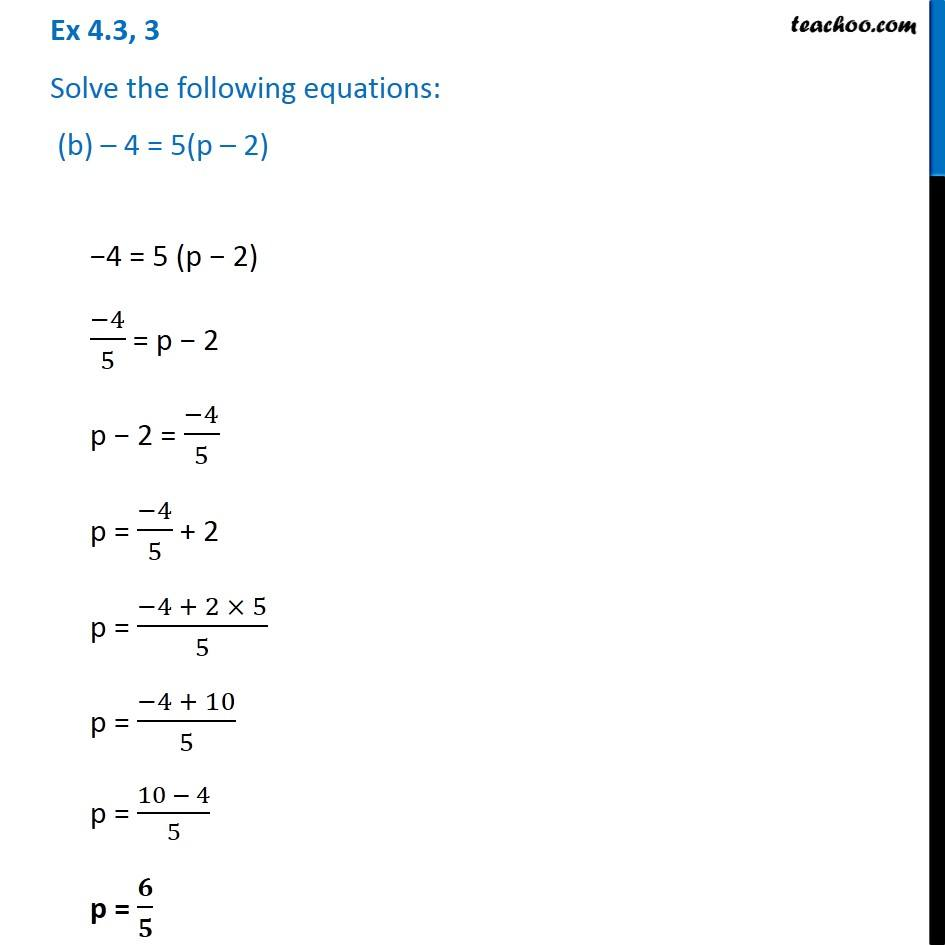1. Chapter 4 Class 7 Simple Equations
2. Serial order wise
3. Ex 4.3

Transcript

Ex 4.3, 3 Solve the following equations: (b) – 4 = 5(p – 2)−4 = 5 (p − 2) (−4)/5 = p − 2 p − 2 = (−4)/5 p = (−4)/5 + 2 p = (−4 + 2 × 5)/5 p = (−4 + 10)/5 p = (10 − 4)/5 p = 𝟔/𝟓